# Shapes of distributions

### Shapes of distributions

#### Lessons

There are two main types of Distribution we are concerned with in statistics:

Frequency Distributions:
A graph representing the frequency of each outcome occurring.

Probability Distributions:
A graph that provides the probability of each outcome occurring.

The most common distribution shapes are:

Symmetric: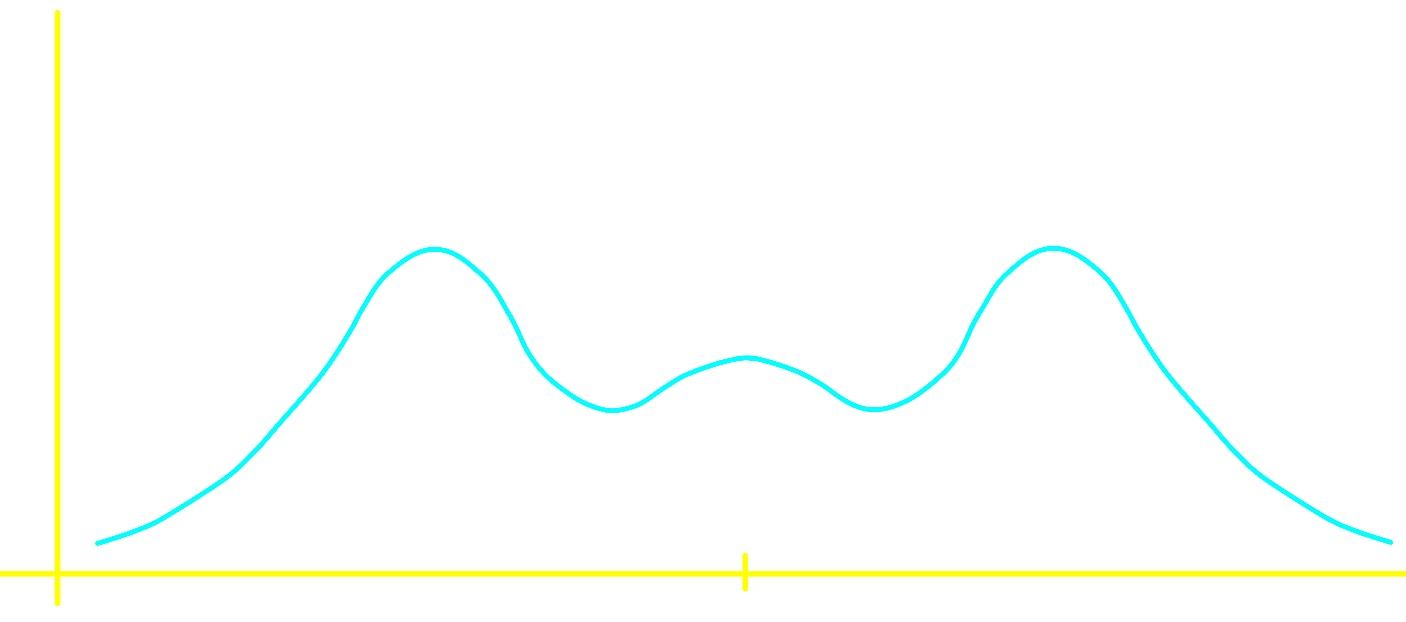Bell-shaped: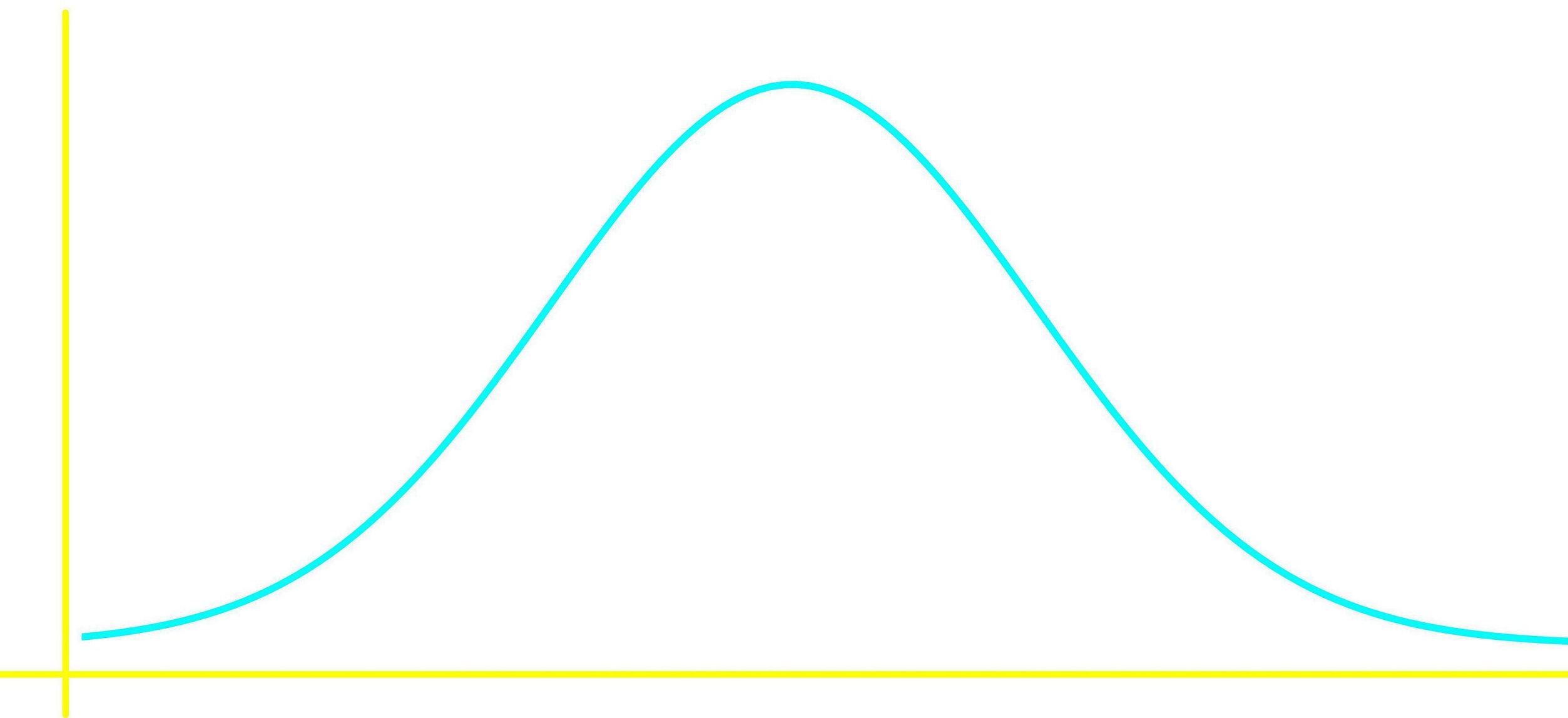Skewed to the left: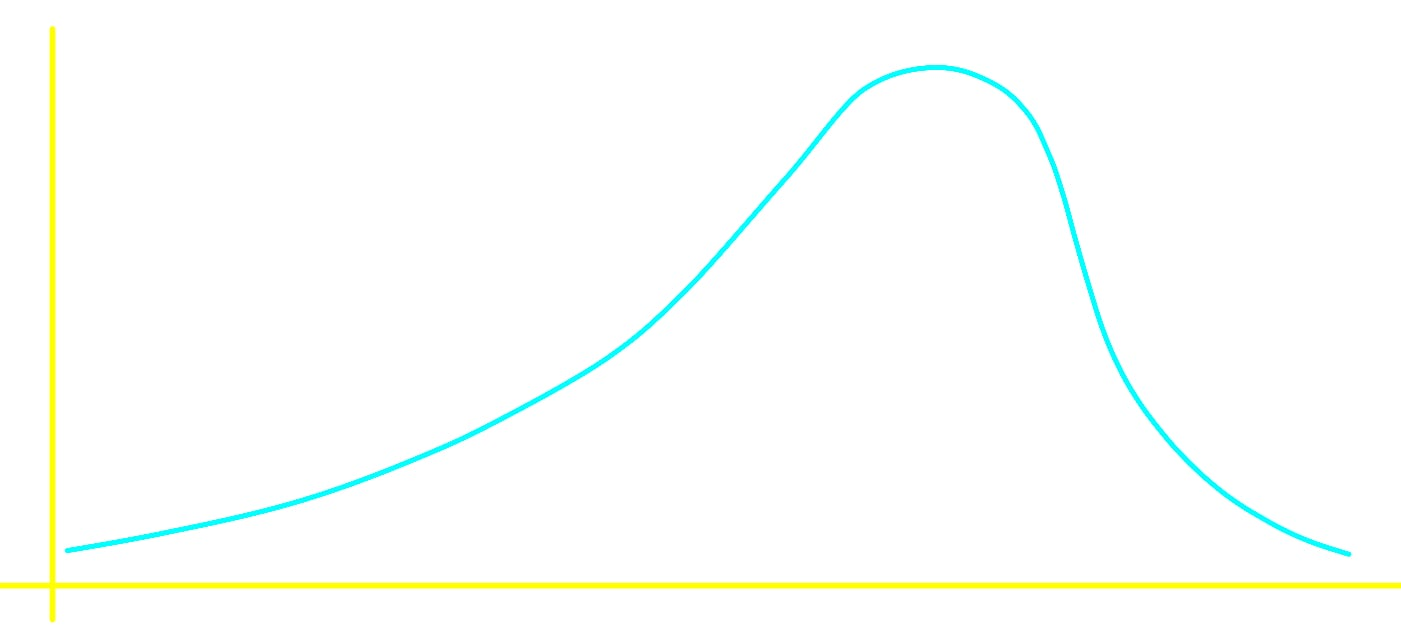Skewed to the right: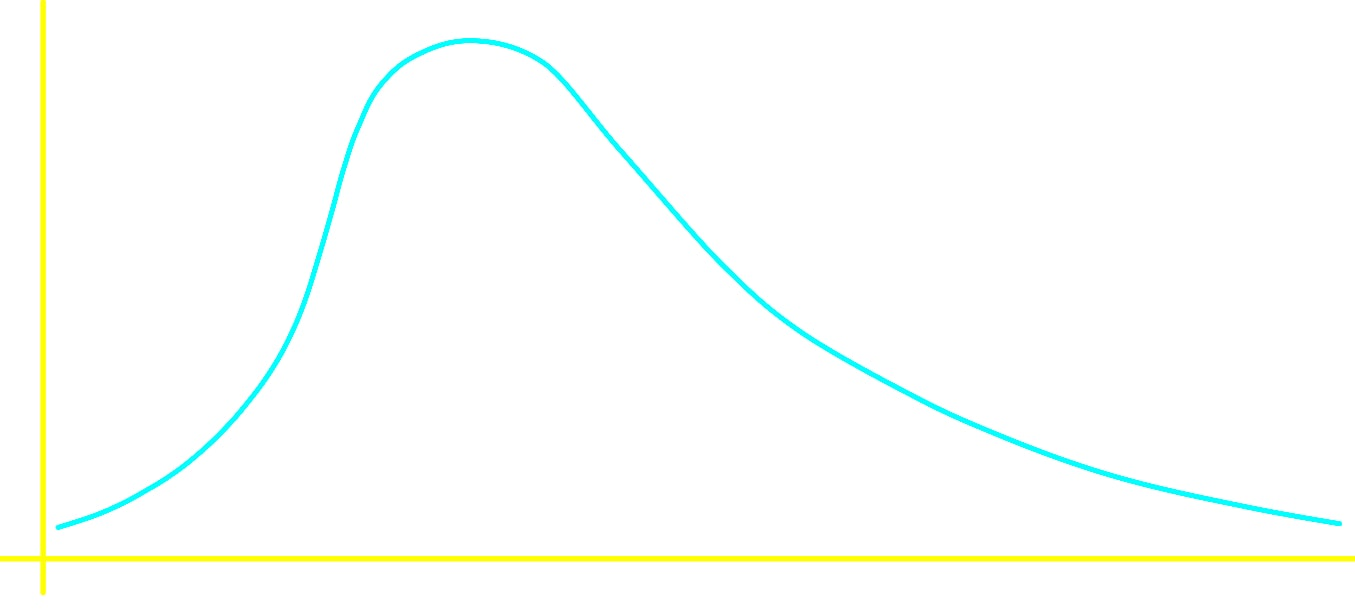Uniform:Cluster:

On a graph a cluster is when several data points lie close together. Typically this means that these data points have similar characteristics, which is also called things are homogeneous (Things which are very diverse or dissimilar are called heterogeneous)

Gap:

A gap is an interval which contains no data.

Peak:

A peak is the highest point of a data set.
• Introduction
a)
All the different ways to arrange data
i.
Symmetric
ii.
Skewed
iii.
Bell-shaped
iv. Uniform

b)
Clusters, gaps and peaks

• 1.
Exploring Different Types of Distributions:
In Mrs. Katsufrakis' class the scores for a test are given in the graph below: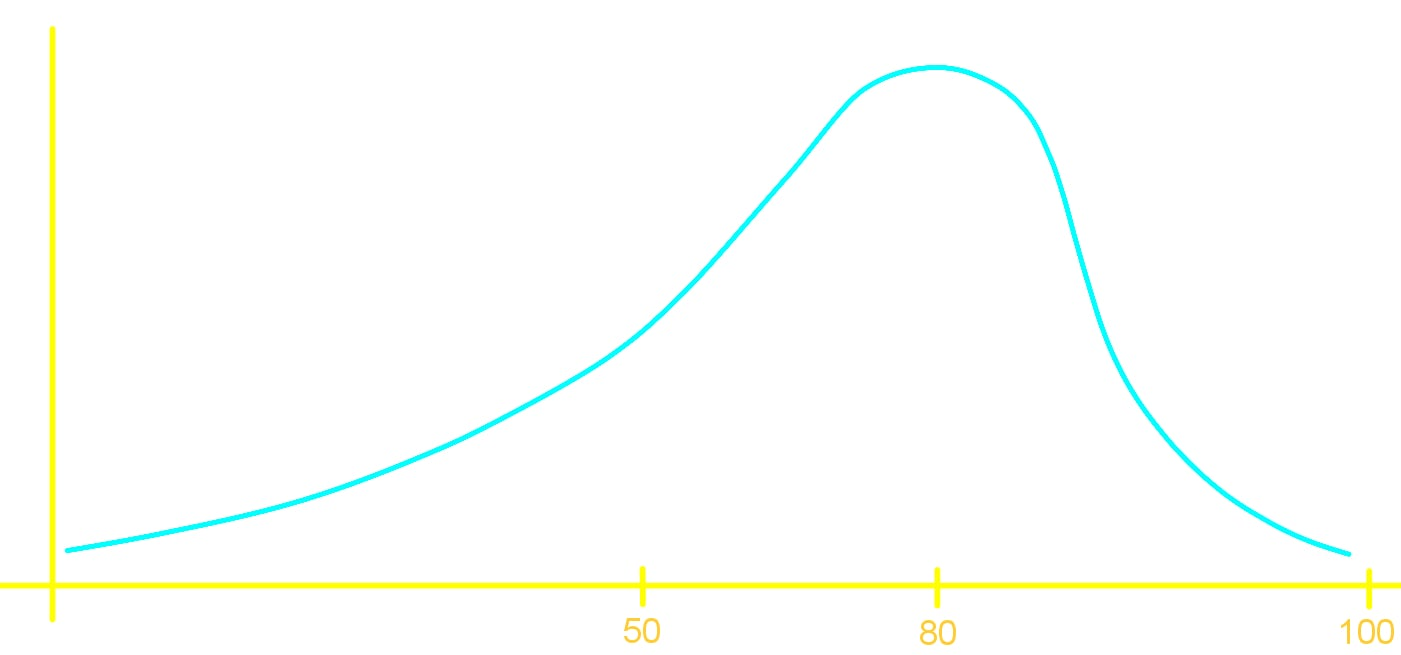What is the average score?
i.
90%
ii.
80%
iii.
70%

• 2.
A bank assures you that one of their tellers will be of assistance to you in 5 minutes or less. Further the bank tells you that the teller will become available equally likely at any time throughout those first 5 minutes. What does the distribution of this wait time look like?

• 3.
An IQ test has an average score of 100 and has more data clumped closer to the mean than further away. What might be the distribution of an IQ test?

• 4.
Clusters, Gaps and Peaks
The finishing times of a marathon are given in the following distribution below: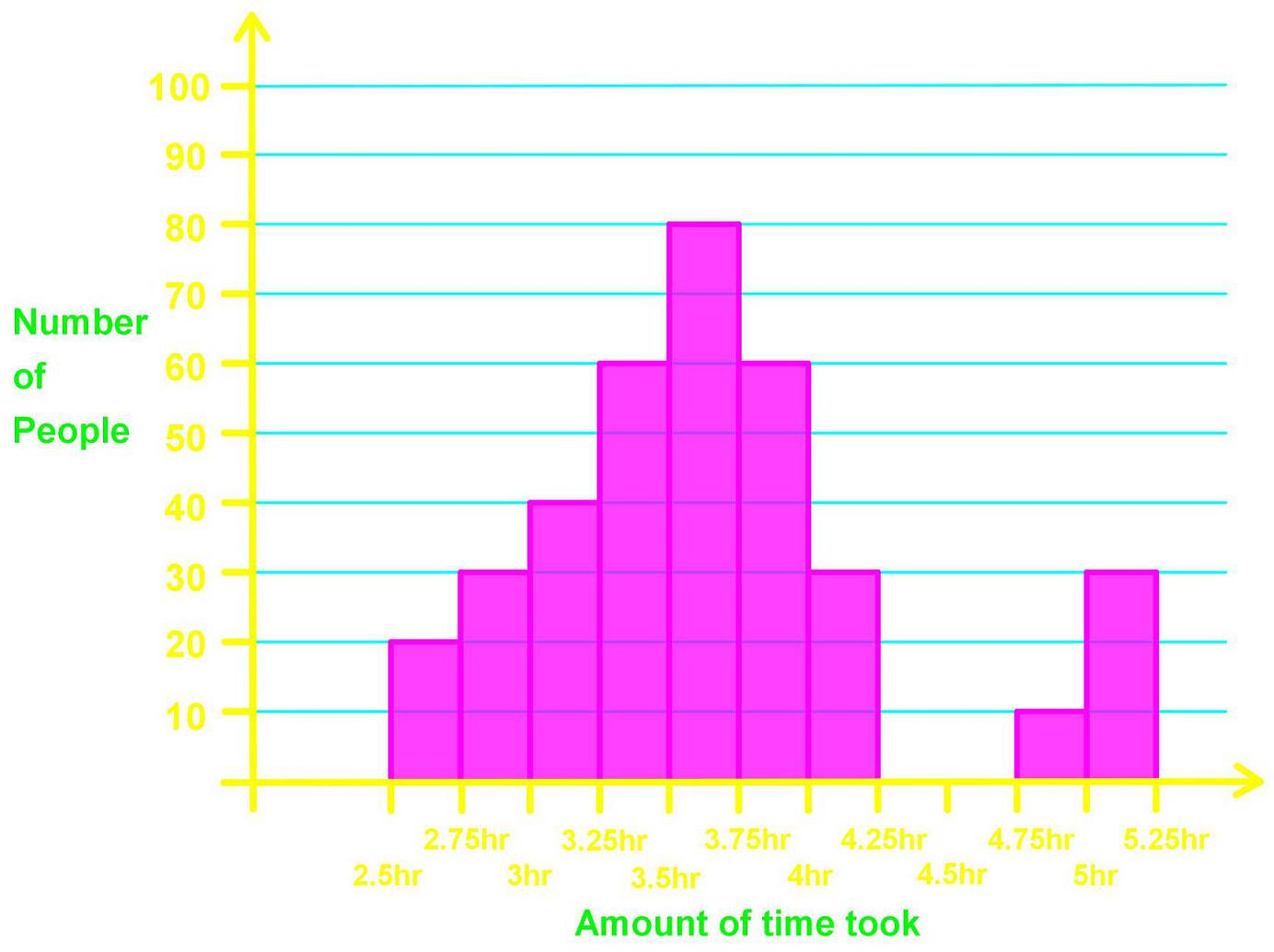Signify where all the clusters, gaps and peaks are.